Mobile QR Code1. (Hameed Majid Advanced Polymeric Materials Research Lab., Physics Department, College of Science, University of Sulaimani, Qlyasan Street, Sulaimani 46001, Kurdistan Regional Government, Iraq )
2. (Department of Civil engineering, College of Engineering, Komar University of Science and Technology, Sulaimani 46001, Kurdistan Regional Government, Iraq)

ZnO semiconductor, band structure, first principle calculation, E-K diagram, optical properties

## I. INTRODUCTION

There are uses of ZnO semiconductors in several fields, including gas sensors, surface acoustic wave devices, transparent contacts, and biomedical materials. The outstanding characteristics of ZnO-based semiconductors are the direct bandgap (BG) of 3.37 eV with a considerable binding energy of exciton. These are well-known suitable photonic materials in ultraviolet and visible regions. The rationalization of designing modern optoelectronic devices relies on direct BG manipulation in an attempt to create barrier layers and quantum wells in device heterostructures . Nowadays, zinc oxide has attracted attention because of an appropriate direct BG of zinc oxide (ZnO) of 3.37 eV, comparable to GaN semiconductor material. At room temperature, ZnO experiences relatively large exciton energy (60 meV) compared to GaN semiconductors [2-4]. The ZnO semiconductor is a well-known material characterized by non-toxicity, low cost, and abundant resources. Fig. 1 shows the three varieties of Zinc oxide crystals: rock salt, zinc blende, and wurtzite structures.

The most prevalent ZnO phase at room temperature and pressure is wurtzite, but the zinc-blende structure can only be stable on cubic substrates. The unit cell in these two structures is a tetrahedron with five atoms; one atom belongs to$~ \mathrm{Zn}^{+2}$ and is surrounded by four $\mathrm{O}^{-2}$, and vice versa . The hexagonal unit cell's lattice parameters measured by X-ray diffraction under ambient circumstances are $c=~ 5.2069\mathrm{A}^{\mathrm{o}},$ $a=~ 3.2495\mathrm{A}^{\mathrm{o}}$, and the density is equal to $5.605\mathrm{g}/\mathrm{cm}^{3}$, and the axial ratio $(c/a$) is equal to $1.603$ [6,7].

The tetrahedral interaction between Zn$^{+2}$ and O$^{-2}$ ions causes polar symmetry along the hexagonal axis. Currently, in optical and mechanical manufacturing of devices, ZnO has widely been utilized , including Light Emitting Diode [9,10], Lasers , optoelectronic devices , photocatalysis , solar cells [14,15], optoelectronic devices , gas sensor . This is owing to the wide BG of ZnO, which provides it weakly susceptible to visible light; thereby, only ultraviolet light has been absorbed. Additionally, its conductivity and the number of carriers are modest .

The doping of transition metal into ZnO enables it to be applicable in optical and mechanical applications. As stated in the literature, various experimental investigations of the optical and structural characteristics of transition metals and vanadium (V) doped ZnO have been documented. S. Maensiri, et al.  dealt previously with the V-doped ZnO in terms of structural and optical properties using the sol-gel method. The study verified that the BG of the V-doped ZnO samples was reduced upon the addition of V. Wang et al.  used both x-ray photoelectron spectroscopy and energy dispersive spectroscopy (EDS) to examine the structural and optical properties of V-doped ZnO. It was established that V-doping causes all films to have a wurtzite structure and grow mostly along the c-axis. The films' transmittance was measured in the calculation of the optical properties. Gherouel et al. , examined the optical and structural characteristics of V-doped ZnO using spray pyrolysis for various controlled concentrations (1-5\%) of V element. The BG energy varied between 3.17 and 3.25 eV, corresponding to the vanadium quantity. Within the 300-1800 nm range of optical transmission and reflectance spectrum for each V-doped ZnO sample, maximum transparency (80-90\%) was recorded in the visible range. Abaira et al. , performed optical and structural characteristics of V-doped ZnO by the sol-gel technique. It was concluded that the doping of V from (2-10\%) makes the BG wide. The study of optical properties has shown that the presence of vanadium widens the optical BG. It is observed that both structural and optical characteristics of doped ZnO samples have extensively been identified from a theoretical standpoint. Changlong Tan et al. , carried out optical and structural characteristics of Cd-doping ZnO. The prediction of the BG of a Cd-doped ZnO monolayer was documented using first-principle DFT in terms of the Hubbard U + local density approximation (LDA) method. Looking at the negative sign of energy formation of the doped sample, it is emphasized that the Cd incorporation sample is chemically stable.

Jianfeng et al.  reported the Cu/Al-doped ZnO systems with optical characterization using the FPC plane-wave pseudopotential method in the generalized gradient approximation in conjunction with the Hubbard U correction based on DFT. Co-doping is the method of choice to reduce the BG of ZnO, consequently causing the absorption edge of the systems to undergo red-shifting and possessing the greatest absorption in the visible light region. To explore the structural, electronic band structure, and optical characteristics of V-doped ZnO, the FPC based on DFT has been implemented in CASTEP . Based on the approach, insight into the optical properties of V doping in ZnO at the microscopic scale is achieved. From the energy calculation, it is predictable to quantify the lattice energy of the V incorporation at the Zn replacement site. The pure ZnO possesses wide BG energy, and the direct BG upon V doping has been obtained. Also, the creation of new levels at the G (gamma) point has been verified, leading to an upward shift of the valence band (VB). Additionally, a substantial BG lowering occurs upon V doping. A state relocation to higher energy can be detected from the density of states diagram. Redshifts as the optical properties of ZnO are observed as a consequence of V doping within-host lattice.

##### Fig. 1. Schematic illustration of (a) rocksalt; (b) zinc blend; (c) hexagonal wurtzite the phase of ZnO structures.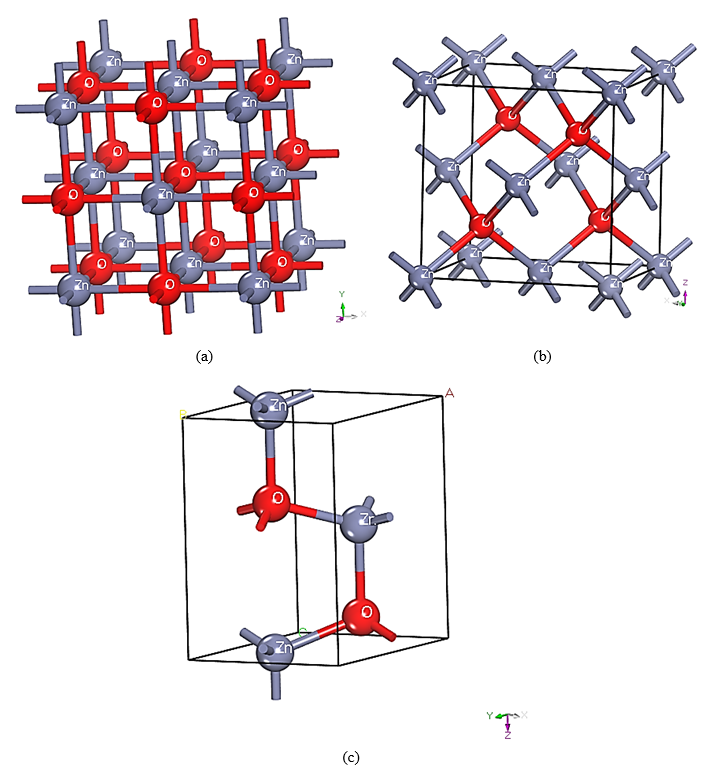## II. THEORETICAL MODELS AND CALCULATION METHODS

### 2.1 Theoretical Models

The hexagonal system is the crystal structure of wurtzite ZnO, with the space group of P63mc. In the structure, each zinc ion is surrounded by four oxygen ions and also the three parameters of the unit cell are as follows: $a=b=3.249\mathrm{A}^{\mathrm{o}}$, $c=5.205\mathrm{A}^{\mathrm{o}}$ . To make the experimental results consistent, the supercells $Zn_{1-x}V_{x}O$, $3\times 2\times 2$, $2\times 2\times 2$, and $2\times 2\times 1$ have been established corresponding to $4.1\%$, $6.2\%$, and $12.5\%$ doping, where V atoms can replace Zn atoms. Fig. 2 shows the proposed supercells.

##### Fig. 2. Designed Models for (a) $\mathrm{ZnO}$; (b)$~ \mathrm{Zn}_{0.9583}\mathrm{V}_{0.04166667}\mathrm{O}$; (c) $\mathrm{Zn}_{0.9375}\mathrm{V}_{0.0625}\mathrm{O}$; (d) $\mathrm{Zn}_{0.875}\mathrm{V}_{0.125}\mathrm{O}$.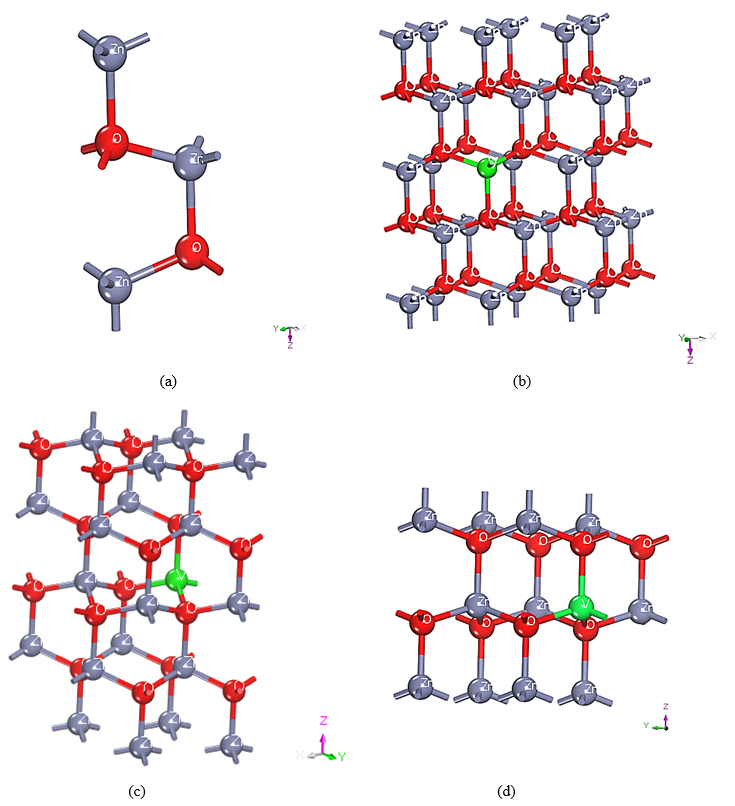### 2.2 Calculation Method

Taking the DFT into consideration and aiming at the plane-wave-pseudo potential (PWP) approach, the first-principles calculations have been carried out using the CASTEP code. The computations were based on DFT, as applied in the Materials Studio package's CASTEP software . The fast calculation is the major feature of the applied approach because of its relatively high efficiency in which, in advances, approximating the shape of the orbitals is absent. The assumption is that the core of the ions is both inner electrons and nuclei; on the other hand, the valence electrons will interact and thus assure fast convergence of ion-electron potential. For electron-ion interaction investigation, the ultrasoft pseudopotential (USP) has been applied in addition to generalized gradient approximation (GGA) and the electron exchange interaction proposed by Perdew-Burke-Ernzerhof (GGA-PBE) [23,24]. The PWP method relies strongly on an assumption of governing almost all physical and chemical characteristics of solids and molecules by valence electrons. This attempt rationalizes the real atoms as pseudo atoms; wherein just the valence electrons are involved in the self-consistent calculation (i.e., neglecting the impact of core electrons in the calculation). Therefore, the cost of computational calculation reduces considerably . The exchange and correlation energy possess impact; herein, it has been dealt with within the GGA . The certain valence electronic configuration used in the current calculation is $\mathrm{Zn}\colon 3d^{10}4s^{2}$, $\mathrm{V}\colon 3d^{3}4s^{2}$, and $\mathrm{O}\colon 2s^{2}2p^{4},$for the materials in a reciprocal space form.

After the self-consistent process, the structure's total energy converges, the force exerted on the atoms is smaller than 0.03 eV/nm, and the stress deviation is less than 0.05 GPa. Just to take into consideration, the K points of the Brillouin zone are $5\times 5\times 4$ ($1\times 1\times 1$ unit cells); $3\times 2\times 3$, ($2\times 2\times 1$, $2\times 2\times 2$, and $3\times 2\times 2$, supercells). The first optimization was the crystal structure, followed by the electronic structure and total and partial densities of state. Afterward, the optical characteristics have been specified using the outcomes obtained from the structural parameters. The accurate description of the electronic structure of oxides doped with transition metal V and localized oxygen components is quantitatively performed from the GGA+U [27,28]. However, apparent limitations of DFT in GGA make the bandgap to be lower than the experimental value (3.37~eV) . Furthermore, the energy calculation for the first Brillouin zone has been carried out by implementing the special k-point sampling techniques of the Monkhorst-Pack scheme . The total energy of different k-point grids has been computed for all compounds to reach a satisfying mesh to ensure the accuracy of the whole results. The electronic structure was modified by GGA+U for all systems in this study, the energy of the 2p level of O and the 3d level of Zn take the values Ud, Zn =10 eV, Us, Zn = 0 eV, Up, Zn = 0, and Up, O = 7 eV , respectively.

## III. RESULTS AND DISCUSSION

### 3.1 Lattice Parameters Study

A common theoretical tool used to determine crystal structure is the geometry optimization procedure. Full geometrical optimizations on the atomic positions or cell parameters are carried out as presented in Table 1. In the theoretical calculations, only a single crystal structural data of the compound of interest have been taken into account. For example, the pure ZnO, ($a=b=3.2492\mathrm{A}^{\circ}$ and $c=5.2054\mathrm{A}^{\circ}$, $c/a=1.602$) found these lattice parameters are quite in agreement with the following experimental values; ($a=b=3.25\mathrm{A}^{\circ}$, $c=5.207\mathrm{A}^{\circ}$, $c/a=1.602$) with the variance of less than $2\%$ . Despite this, the predicted lattice parameters and unit cell volumes were somewhat out compared with the experimental findings published in the literature (within 2 percent). On the other hand, satisfactory agreement with the existing theoretical [17, 27, 30, 31] findings has been attained. It's worth noting that the GGA approximation has a built-in tendency to overestimate lattice parameters. As long as the computations are as accurate as they need to be, these types of mistakes are acceptable. Another interesting feature of the data is the tiny amount of variance between the theoretical and experimental values. First-principles computations are accurate as a consequence. The volume and lattice parameters of the ZnO system in the a and c axes increase as the quantity of V$^{5+}$ ions in doping is increased. The doped system's lattice properties and volume are somewhat higher than those of the parent ZnO system. This is caused by the indigence to calculate the electronic structure properties in which the lattice parameters are impacted.

In calculating the BG of most systems, the limitations involved in the GGA approximation, make the BG calculations to be as not reached the level of reflecting the actual results. Another cause of the present results is the repulsive interactions of the extra electrons of the V$^{5+}$ ion that exists effectively. As a result of both factors' impact, the volume of the doped sample rises as the amount of V-doping increases. And also in Table 1. Different doping elements present various impacts on the changing rate of the lattice parameters a and c. and the ratio between them floats up and down.

##### Table 1. The lattice parameters of pure ZnO and doped ZnO for different elements after geometry optimization
 Structure a=b/A˚ c/A˚ V/$\mathrm{A˚}^{3}$ Ref. ZnO ZnO: In O-rich Zn-rich 3.298 3.295 3.295 5.318 5.319 5.319 50.0 50.1 50.1  ZnO ZnO: Ag AL 3.249 3.238 5.206 5.202  ZnO ZnO: Al Cu 3.286 3.278 5.283 5.290 49.401 49.239  ZnO ZnO:V 2% ZnO:V 6% ZnO:V 10% 3.247 3.261 3.235 3.250 5.202 5.326 5.283 5.210  ZnO ZnO:S ZnO:N ZnO:S N 3.242 3.272 3.248 3.262 5.210 5.250 5.231 5.255  ZnO ZnO: Cu 2.78 % ZnO: Cu 4.17% 3.249 3.249 3.272 5.205 5.205 5.284 54.9 54.9 61.2  ZnO ZnO:Fe ZnO:Cu ZnO :Cd ZnO:B ZnO:N ZnO:S 3.326 3.335 3.326 3.358 3.312 3.333 3.373 5.420 5.396 5.417 5.456 5.582 5.433 5.515  ZnO ZnO:A ZnO:Mo ZnO:Mo ,A 3.25 3.247 3.275 3.270 5.23 5.232 5.237 5.249 57.385 57.326 58.374 58.327  ZnO ZnO: Fe ZnO: Co 3.288 3.296 3.295 5.307 5.274 5.267 198.863 198.285 198.180  ZnO ZnO:V 4.16% ZnO:V 6.25% ZnO:V 12.5% 3.2492 3.25655 3.26164 3.28078 5.2054 5.2402 5.2530 5.2870 47.5948 48.1270 48.3915 49.2810 Present paper

### 3.2 Band Structure and Density of State Studies

The work conducted in this research specified the band structure along a high symmetry direction of the Brillouin zone (G-A-H-K-G-M-L-H) for pure ZnO and V-doped ZnO. When determining a material's characteristics, it is crucial to accurately determine its density of states (DOS) and its bandgap structure. In addition, the likelihood of electrons occupying certain energy ranges may be calculated from the shape of the electronic band (that is, energy bands). As a result, the bandgap refers to the unusable energy ranges. The electronic BG may be measured in insulators and semiconductors by comparing the valence band maximum (VBM) with the conduction band minimum (CBM). The electronic band structure computations will be useful in determining the probable transition of electrons from VBM to CBM. Intuitively, the valence band lies below the Fermi state $\left(E_{F}\right)$ at 0 eV, and the CB locates above the BG energy.

From Fig. 3, a direct G-to-G transition BG energy of 3.331 eV for super-cells including, $\mathrm{Zn}_{0.9583}V_{0.04166667}O$ and $\mathrm{Zn}_{0.9375}V_{0.0625}O$ and the band structure calculated along the symmetry direction of the Brillion zone (G-A-H-K-G-M-L-H) are recorded. Located at the G point, the valence and conduction bands have a straight $G-to-G$ transition. This suggests the existence of p-type semiconductors of $\mathrm{Zn}_{0.9583}V_{0.04166667}O$ and $\mathrm{Zn}_{0.9375}V_{0.0625}O$. It is principally accepted that the direct nature of the BG contains a transition of an electron from the valence band to the conduction band without phonons assistance which is mostly free of losing incident energy during transitions. The bottom 10 bands (occurring around -9 eV) are related to $\mathrm{Zn}\colon 3d$ levels. The next 6 bands from -5 eV to 0 eV correspond to $\mathrm{O}\colon 2p$ bonding states [33,34]. Based on the DFT approach obtained in the current work it is possible to design doped ZnO with direct BG. The BG energy was reduced from 3.331 eV for neat ZnO to 2.055 eV for ZnO dopped with 12.5 percent of V atom. Ultimately, the direction of the Brillouin zone was (G-A-H-K-G-M-L-H), and the direct $G-to-G$ transition of the supercell $\mathrm{Zn}_{0.875}V_{0.125}O,$ evidence formation of p-type semiconductors.

This model tells that the BG can be reduced to the energy of $2.055\mathrm{eV}$. Fig. 3(a) demonstrates that the value of the direct band in parent ZnO is lower than that of the experimental value (3.37 eV).In other words, the theoretical values of the BG energy are lower than the experimental values in all cases. This might be due to the lack or poor description of strong Coulomb correlation and exchange interaction between electrons. Notably, the prominent notice is that the calculated BG of the present and previous studies are in good agreement, as presented in Table 2.

The DOS and partial density of states (PDOS) of both parent $ZnO$, $\mathrm{Zn}_{0.9583}V_{0.04166667}O$, $\mathrm{Zn}_{0.9375}V_{0.0625}O$, and $\mathrm{Zn}_{0.875}V_{0.125}O$ supercells are plotted and exhibited in Fig. 4. Overall, introducing the V atoms into the lattice results in existing of primarily $\mathrm{O}\colon 2s^{2}2p^{4}$ state, $\mathrm{V}\colon 3\mathrm{d}^{2}$ in the top of valence, forming $V-O$ bonds besides $Zn-O$ bonds. In Fig. 4, PDOS was applied to analyze the electronic structure deeply providing a contribution of the energy bands in a specific atomic orbital.

In parent ZnO, both $\mathrm{Zn}\colon 3d^{10}4s^{2}$, and $\mathrm{O}\colon 2s^{2}2p^{4}$ states contribute mainly to the valance bands between ${-}$5.5 and 0 eV, and $\mathrm{Zn}\colon 3d^{10}4s^{2}$, $\mathrm{V}\colon 3d^{3}4s^{2}$, and $\mathrm{O}\colon 2s^{2}2p^{4}$ states are involved in the conduction bands ranging between 3.318 and 12.4 eV. For super-cells, there are additional contributions of $\mathrm{V}\colon 3d^{3}4s^{2}$ states. The localized $\mathrm{V}\colon 3d^{2}$ energy energy state substitutes dopant V ions for Zn, localizing within the Fermi level between -0.7 and 0.5 eV. The presence of $\mathrm{V}\colon 3d^{3}4s^{2}$ also also within, the energy gap from 1.6 to 3 eV reduces the energy gap. The existence of these states between 4 to 8~eV is evidenced. Consequently, the PDOS of all these states increases with increasing the quantity of V in the doping process.

##### Fig. 3. The E-K (Band Structure) diagram for (a) ZnO; (b) $\mathrm{Zn}_{0.9583}\mathrm{V}_{0.04166667}\mathrm{O}$; (c) $\mathrm{Zn}_{0.9375}\mathrm{V}_{0.0625}\mathrm{O}$; (d) $\mathrm{Zn}_{0.875}\mathrm{V}_{0.125}\mathrm{O}$.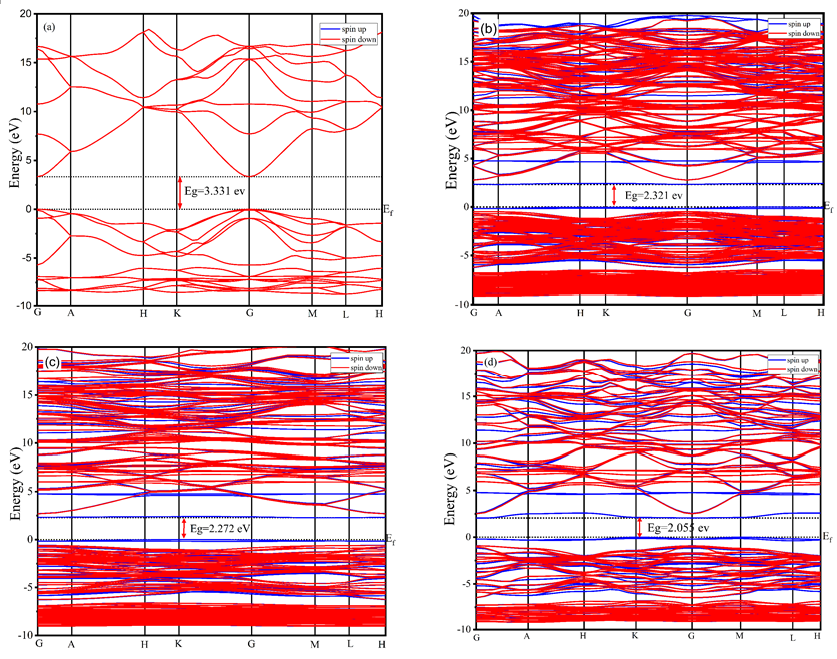##### Fig. 4. The PDOS and total DOS for (a) ZnO; (b) $\mathrm{Zn}_{0.9583}\mathrm{V}_{0.04166667}\mathrm{O}$; (c) $\mathrm{Zn}_{0.9375}\mathrm{V}_{0.0625}\mathrm{O}$; (d) $\mathrm{Zn}_{0.875}\mathrm{V}_{0.125}\mathrm{O}$.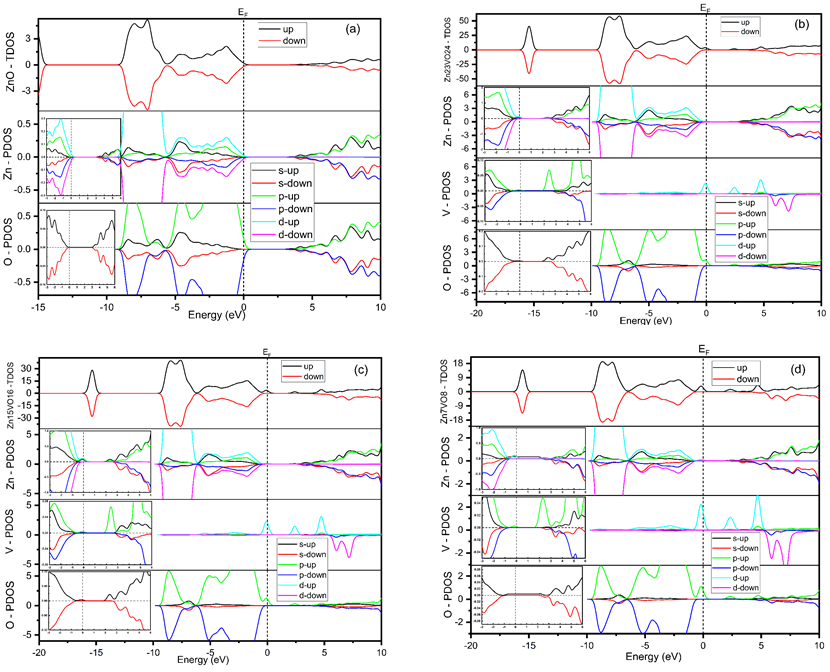##### Table 2. The direct bandgap energy of pure ZnO and doped ZnO for different elements after geometric parameters optimization
 Structure Eg/eV Functional Computer code Ref. ZnO ZnO: In 5.55% 3.40 2.91 GGA+U Castep  ZnO ZnO: Cu 0.241 0.295 GGA Castep  ZnO:V 1% ZnO:V 2% ZnO:V 3% ZnO:V 4% ZnO:V 5% 3.17 3.23 3.25 3.20 3.22 Experimental  ZnO 4.0 LDA+U Castep  ZnO ZnO:Cu ZnO:Al ZnO:Cu Al 3.322 3.101 3.086 3.035 GGA+U Castep  ZnO ZnO:Al ZnO:Ag ZnO:Ag Al 3.309 3.201 2.878 2.986 GGA+U Castep  ZnO ZnO:Al ZnO:Ag ZnO:Ag Al 0.745 0.673 0.502 0.204 GGA ZnO ZnO: Cu 2.78% ZnO: Cu 4.17% 3.373 1.122 1.031 GGA+U Castep  ZnO ZnO:Fe ZnO:Cu ZnO:Cd ZnO:B ZnO:N ZnO:S 0.80 1.45 0.48 0.65 0.59 0.85 0.44 GGA Castep  ZnO ZnO: Fe ZnO: Co 0.735 1.107 1.04 GGA Castep  ZnO ZnO: Ag ZnO: Co 3.4 2.8 3.24 GGA+U Castep  ZnO ZnO:V 4.16% ZnO:V 6.25% ZnO:V 12.5% 3.331 2.321 2.272 2.055 GGA+U Castep Present work

### 3.3 Optical Properties

Crystals' band structure and optical qualities are reflected in the dielectric function, which may be considered the point at which electronic transition and electronic structure meet. The solid-state technique states that the complex dielectric function $\varepsilon \left(\omega \right)=\varepsilon _{1}\left(\omega \right)+i\varepsilon _{2}\left(\omega \right)$ can express the optical properties of semiconductor materials . On the one hand, the imaginary part results from transitions between the CB and the VB. Calculating momentum matrix components for filled and non-filled levels and Kramers-Kronig dispersion relations theoretically yields both the real $\varepsilon _{1}\left(\omega \right)$ and imaginary $i\varepsilon _{2}\left(\omega \right)$ portions of dielectric functions, respectively [36,37]. According to quantum mechanics, the system's photon-electrons interact through time-dependent disturbances in the system's ground electronic state. The absorption or emission of photons triggers transitions between occupied and unoccupied states in a quantum system. In terms of DOS, excitation spectra may be thought of as a conduction-valence band DOS . Furthermore, from $\varepsilon _{1}\left(\omega \right)$ and $i\varepsilon _{2}\left(\omega \right)$, extraction of other optical properties is obtainable. The optical properties from the density functional theory are achievable, for instance, the dielectric function, absorption coefficient, and refractive index  equation (1,2,3,4,5) [12, 31, 38-41].

##### (1)
$$\varepsilon _{1}\left(\omega \right)=\frac{2}{\pi }\rho _{0}\int _{0}^{\infty }\frac{\omega '\varepsilon _{2}\left(\omega \right)}{\omega '^{2}-\omega ^{2}}d\omega$$
##### (2)
$\varepsilon _{2}\left(\omega \right)=\frac{C}{\omega ^{2}}\sum _{V,C}\int _{BZ}\frac{2}{\left(2\pi \right)^{3}}\left| M_{CV}\left(k\right)\right| ^{2}.\delta \left(E_{C}^{k}-E_{V}^{k}-\mathrm{\hslash }\omega \right)d^{3}k$
##### (3)
$\alpha \left(\omega \right)=\sqrt{2}\omega \left[\sqrt{\varepsilon _{1}^{2}\left(\omega \right)+\varepsilon _{2}^{2}\left(\omega \right)}-\varepsilon _{1}\left(\omega \right)\right]^{\frac{1}{2}} \\$
##### (4)
$n\left(\omega \right)=\left[\sqrt{\varepsilon _{1}\left(\omega \right)^{2}+\varepsilon _{2}\left(\omega \right)^{2}}+\left(\omega \right)\right]^{\frac{1}{2}}/\sqrt{2} \\$
##### (5)
$k\left(\omega \right)=\left[\sqrt{\varepsilon _{1}\left(\omega \right)^{2}+\varepsilon _{2}\left(\omega \right)^{2}}-\left(\omega \right)\right]^{\frac{1}{2}}/\sqrt{2}$

where $~ \mathrm{\hslash }\omega$ is a photon energy, $k$ is the inverted lattice, respectively. $\omega ~$ and $\rho _{0}$ are frequency and the density of the medium, respectively. The$\delta \left(E_{C}^{k}-E_{V}^{k}-\mathrm{\hslash }\omega \right)$ represents the delta function imposes energy conservation in the third step corresponding to the electron's escape through the crystalline surface. $\left| M_{CV}\left(k\right)\right| ^{2}$ refers to the momentum transition matrix element between initial and final states. $E_{V}^{k,}$ and $E_{C}^{k}$ are the VBM and CBM energy, respectively.

Fig. 5(a) Show that pure ZnO and V-doped systems ($\mathrm{Zn}_{0.9583}V_{0.04166667}O$; $\mathrm{Zn}_{0.9375}V_{0.0625}O$; $\mathrm{Zn}_{0.875}V_{0.125}O$.) have static dielectric constants of around 2.302, 2.553, 2.572, and 2.657, respectively. The band gap is inversely proportional to the $\varepsilon _{1}\left(0\right)$. As a result, the decrease in the band gap can be attributed to the rise in the static dielectric constant, which is consistent with the results of band structure calculations. The band illustrates that V-doped ZnO has a metallic characteristic, yet the $\varepsilon _{1}\left(\omega \right)$ shows that it is dielectric. $\varepsilon _{1}\left(\omega \right)$ is fluctuated between 0 to 12 eV after that it decreases rapidly to -0.3 at 17.4~eV. Evidence of poor light transmission and energy losses within the media with significant reflection can only be obtained using the negative component of the dielectric constant $\varepsilon _{1}\left(\omega \right)$.

The imaginary part of electronic transitions between occupied and unoccupied states accounts for the peaks of $\varepsilon _{2}\left(\omega \right)$. In Fig. 5(b), we can see that there are three distinct peaks of the $\varepsilon _{2}\left(\omega \right)$ for pure and V-doped ZnO. ZnO's DOS and energy band structure interpretation suggests that the peak at 9.5 eV is mostly the optical response to the transition between the $\mathrm{O}\colon 2p$ (higher valence band) and $\mathrm{Zn}\colon 3d$ (lower conduction band) states. The transitions in the valence band, which are between $\mathrm{Zn}\colon 3d$ and $\mathrm{O}\colon 2p$ states, give rise to the peak at 13.17 eV. Transitions between$~ \mathrm{~ Zn}\colon 4d$ (lower valence band) and $\mathrm{O}\colon 2s$ (lower conduction band) states produce a peak of about 16 eV. The electronic transition between the $V\colon 3d$ states in the higher valence band and the $Zn\colon 3d$ levels in the lowest conduction band produces the first peak of about 5 eV near the energy gap. At the high energy range, the remaining three primary peaks stay in their original locations, indicating that there has been no transition.

It is notable, however, that just the first primary peak has altered its location, indicating that ZnO's low-energy area has been effectively doped. However, even if the final peak is due to a mixed transition, it is seen that the loaded sample not only moves absorption peaks to lower energies but also reduces the sharpness of their appearance. Fig. 5 indicates that the BG has narrowed dramatically, resulting in a reduced absorption edge. V-doped ZnO, on the other hand, results in a decrease in energy BG. The red arrow intersection on photon energy (Fig. 5(b)) gives the energy BG (3.32). It is close enough to that estimated (3.331) from the E-K diagram (Fig. 3(a)) for pure ZnO.

The optical absorption spectrum is the vital tool that is mainly focused on. The absorption coefficients of ZnO correspond to various quantities of V are presented in (Fig. 6). The absorption edge value is recorded as 0.68~eV, (in agreement with the paper  for pure ZnO, and there are three distinct absorption peaks at 9.89 eV, 13.5~eV, and 16.12 eV (10.07 eV, 16.87 eV, and 20.69~eV) respectively. The last two peaks are created by the electronic transition between the O-2p state and Zn-4s state. A red shift is seen in the absorption of doped ZnO with V compared to parent ZnO at the lower energy state, originating from the BG reduction. The parent ZnO is not a strong absorber in the IR region; however, it is a good absorber in the UV region. It is well-known that the energy of visible light lies between 1.62 and 3.11 eV. From (Fig. 6) we can see that V-doped ZnO has an intense absorption in the ultraviolet region. The right side of (Fig. 6) shows that the pure and V-doped ZnO has a weak absorption in the visible and infrared region. The intense absorption in the visible and near-ultraviolet region is because of the electronic interband transitions in the V-3d states. The electronic intraband transitions in V-3d and Zn-4s states in the conduction band can cause a weak absorption in the infrared region (lower photon energy) . The system has intense absorption at (13.5~eV-16.59 eV) for pure and V-doped ZnO. The absorption peaks indicate that V-doped ZnO could be a good choice for lasers, detectors, or diodes in the infrared and vacuum ultraviolet regions.

The refractive index $n\left(\omega \right)$ is an important optical characteristic that allows material transparency to be measured. The calculated refractive index $n\left(\omega \right)$ and extinction coefficient $k\left(\omega \right)$ of undoped, V-loaded ZnO are shown in (Fig. 7). In (Fig. 7(a)) the $n\left(0\right)$ of parent ZnO is around 1.51 and becoming 1.59, 1.60, and 1.62 for ($\mathrm{~ Zn}_{0.9583}V_{0.04166667}O$; $\mathrm{Zn}_{0.9375}V_{0.0625}O$;$\mathrm{Zn}_{0.875}V_{0.125}O$), respectively after doping. The refractive index in the visible region increases with increasing V concentration. The refractive indices are smaller in the deep ultraviolet band (8-11 eV). Because electrons in the deep level orbital are sheltered by electrons in the shallow level during the transition, electrons in the deep level orbital have a harder time transitioning in the orbit, resulting in a lower refractive index. when it shifts to the higher energy level the refractive index becomes 0.65 at 18.5 eV. As demonstrated in (Fig. 5(b)) the computed imaginary part $\varepsilon _{2}\left(\omega \right)$ of the dielectric constant of undoped and V-doped ZnO are similar. The extinction coefficient $k\left(\omega \right)$ for $\mathrm{Zn}_{0.875}V_{0.125}O$ at the first increase slowly with rising the photon energy but parent ZnO, $\mathrm{Zn}_{0.9375}V_{0.0625}O$ and $\mathrm{Zn}_{0.9583}V_{0.04166667}O$ remains unchanged. We can use the $k\left(\omega \right)$ to determine the energy band gap of the systems.

##### Fig. 5. (a) Real part of Dielectric Function $\varepsilon _{1}\left(\omega \right)$; (b) Imaginary part of Dielectric Function.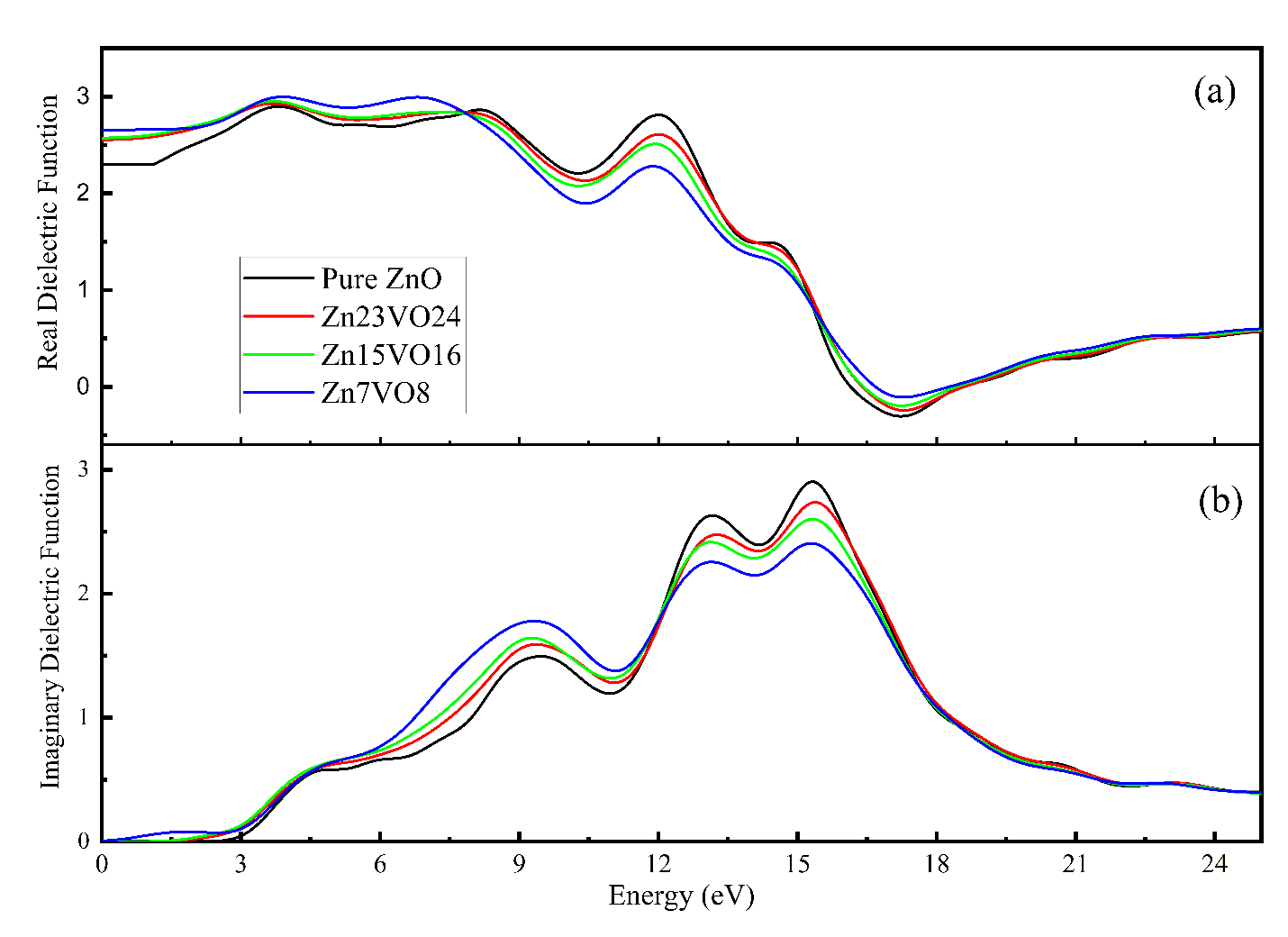##### Fig. 6. Absorption coefficient of pure and V-doped ZnO.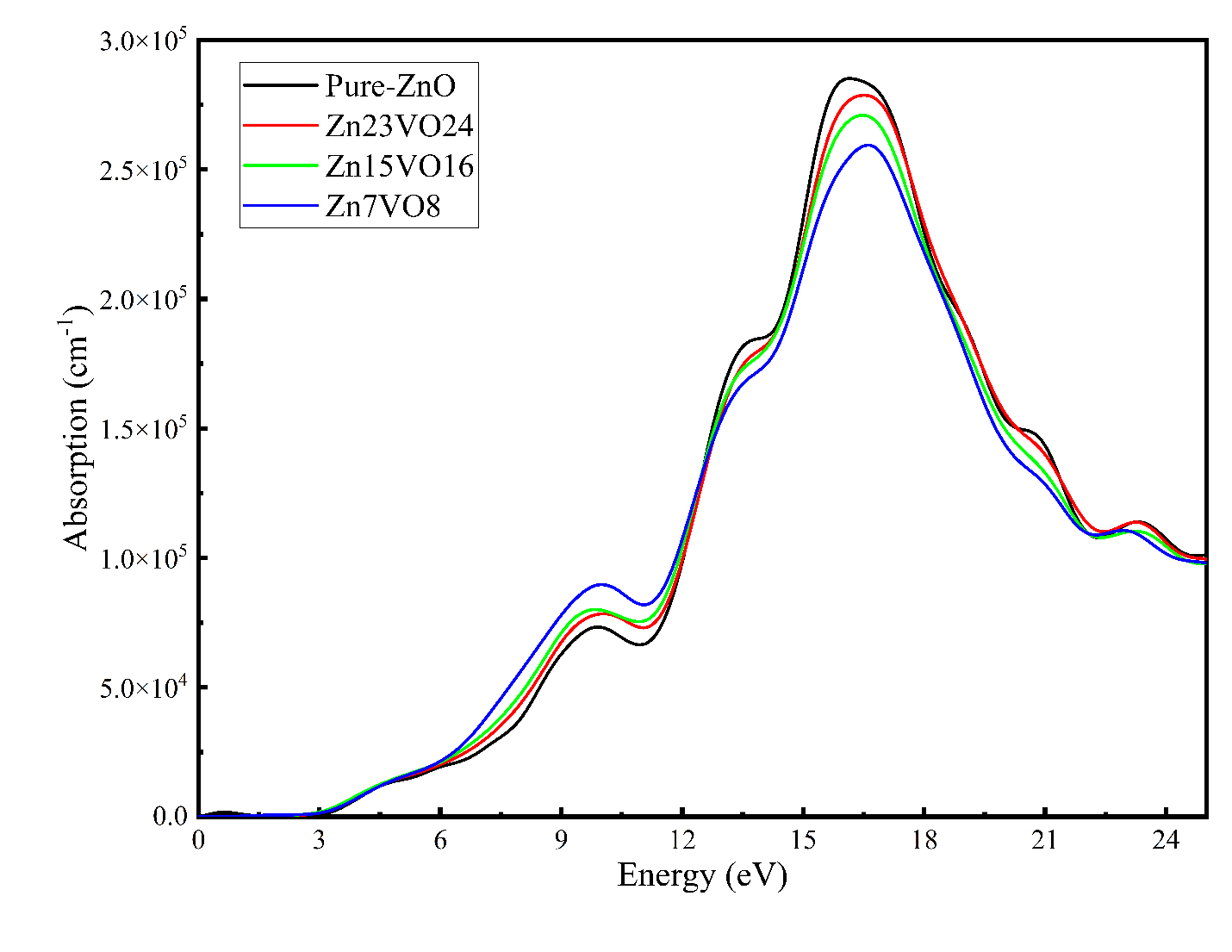##### Fig. 7. Refractive index (a) n(${\upomega}$) and (b) extinction coefficient k(${\upomega}$), for pure and v-doped ZnO with different contents.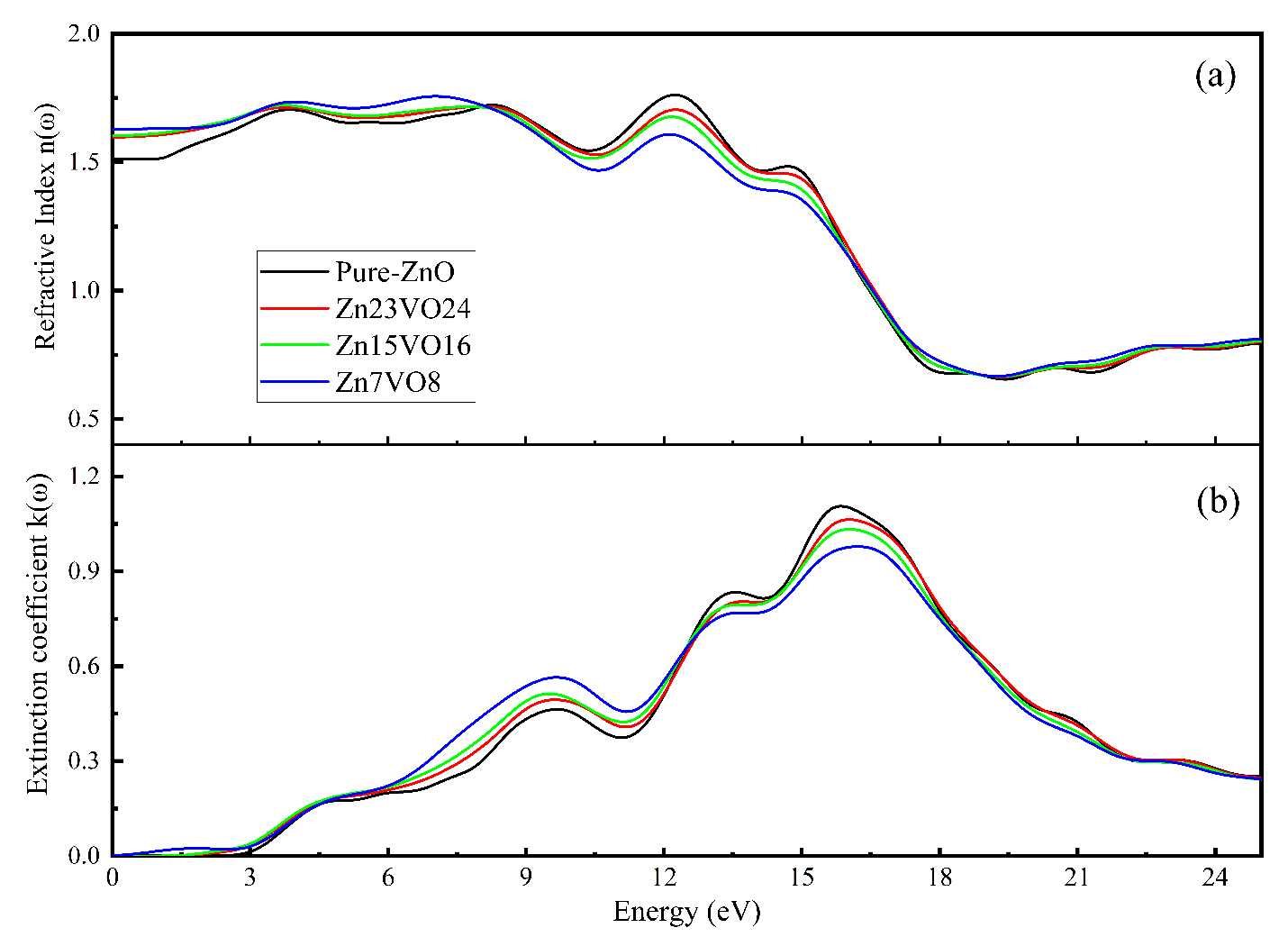## IV. CONCLUSIONS

In conclusion, a first-principles implementation is necessary to assess the structural, E-K diagram, and optical features of the neat and V-doped ZnO systems. According to the calculated results, the BG of the pure and doped systems has dropped from 3.318 to 2.055 eV due to the higher V amount. Increases in V have modified the optical transition between the VBM and CBM. Due to the doping of V, the refractive index, extension coefficient, real and imaginary part dielectric function, and real and imaginary part dielectric function of ZnO are all substantially high in the low energy region. Increased V doping content in ZnO promotes ZnO volume growth. The redshift of the absorption spectrum is enhanced in the IR region when the V content has been increased as an impurity.

## ACKNOWLEDGMENTS

The authors gratefully acknowledge the financial support for this study from the Ministry of Higher Education and Scientific Research-Kurdish National Research Council (KNRC), Kurdistan Regional Government/Iraq. The financial support from the University of Sulaimani is greatly appreciated.

## CONFLICTS OF INTEREST

The authors declare no conflict of interest

## References

1
Ishihara J., Nakamura A., Shigemori S., Aoki T., Temmyo J., 2006, Zn1-xCdxO systems with visible bandgaps, Appl. Phys. Lett., Vol. 89, No. 9, pp. 10-122
Hou Q., Xi D., Li W., Jia X., Xu Z., 2018, First-principles research on the optical and electrical properties and mechanisms of In-doped ZnO, Phys. B Condens. Matter, Vol. 537, pp. 258-2663
Fan Z., Lu J. G., 2005, Zinc oxide nanostructures: Synthesis and properties, J. Nanosci. Nanotechnol., Vol. 5, No. 10, pp. 1561-15734
Song D. M., Wang T. H., Li J. C., 2012, First principles study of periodic size dependent bandgap variation of Cu doped ZnO single-wall nanotube, J. Mol. Model., Vol. 18, No. 12, pp. 5035-50405
Janotti A., Van De Walle C. G., 2009, Fundamentals of zinc oxide as a semiconductor, Reports Prog. Phys., Vol. 72, No. 126
Chuanhui Xia , Feng Wang , Chunlian Hu , 2014, Theoretical and experimental studies on electronic structure and optical properties of Cu-doped ZnO, Journal of Alloys and Compounds, Vol. 589, pp. 604-6087
Sekar A., Kim S. H., Umar A., Hahn Y. B., 2005, Catalyst-free synthesis of ZnO nanowires on Si by oxidation of Zn powders, J. Cryst. Growth, Vol. 277, No. 1-4, pp. 471-4788
Sahal M., Hartiti B., Ridah A., Mollar M., Marí B., 2008, Structural, electrical and optical properties of ZnO thin films deposited by sol-gel method, MicroelectronicsJ., Vol. 39, No. 12, pp. 1425-14289
Gao W., Li Z.W., 2008, Photoluminescence properties of ZnO films grown by wet oxidation: Effect of processing, Journal of Alloys and Compounds10
Luan Z., Sun D., Tan C., Tian X., Huang Y., 2017, First-principles calculations of electronic structure and optical properties of Be-doped ZnO monolayer, Integr. Ferroelectr., Vol. 179, No. 1, pp. 84-9411
El Ghoul J., Bouguila N., Gómez-Lopera S. A., El Mir L., 2013, Structural and optical properties of nanoparticles (V, Al) co-doped ZnO synthesized by sol-gel processes, SuperlatticesMicrostruct., Vol. 64, pp. 451-45912
Chen H., Qu Y., Sun L., Peng J., Ding J., 2019, Band structures and optical properties of Ag and Al co-doped ZnO by experimental and theoretic calculation, Physica E: Low-Dimensional Syst. Nanostructures, Vol. 11413
Wang Q. B., Zhou C., Wu J., Lü T., 2013, A GGA+U study of the optical properties of vanadium doped ZnO with and without single intrinsic vacancy, Opt. Commun., Vol. 297, pp. 79-8414
Gherouel D., Dabbous S., Boubaker K., Amlouk M., 2013, Vanadium doping patterns in ZnO lattices in the lattice compatibility theory framework, Mater. Sci. Semicond. Process., Vol. 16, No. 6, pp. 1434-143815
Tan C., Sun D., Xu D., Tian X., Huang Y., 2016, Tuning electronic structure and optical properties of ZnO monolayer by Cd doping, Ceram. Int., Vol. 42, No. 9, pp. 10997-1100216
Xia C., Wang F., Hu C., 2014, Theoretical and experimental studies on electronic structure and optical properties of Cu-doped ZnO, J. Alloys Compd., Vol. 589, pp. 604-60817
Tan H., Jia C., Ge X., Xiang C., 2012, First-principles conductance calculations of Ag-Al Co-doped ZnO, Adv. Mater. Res., Vol. 468-471, pp. 1726-173118
Xiang Q., Xiong Z., Chen L., Jiang Y., 2012, Effect of Er doping on the electronic structure and optical properties of ZnO, 6th Int. Symp. Adv. Opt. Manuf. Test. Technol. Des. Manuf. Test. Smart Struct. Micro- Nano-Optical Devices, Syst., Vol. 8418, pp. 84181G19
Maensiri S., Masingboon C., Promarak V., Seraphin S., 2007, Synthesis and optical properties of nanocrystalline V-doped ZnO powders, Opt. Mater. (Amst)., Vol. 29, No. 12, pp. 1700-170520
Li-Wei W., Zheng X., Li-Jian M., Teixeira V., Shi-Geng S., Xu-Rong X., 2009, Influence of concentration of vanadium in Zinc oxide on structural and optical properties with lower concentration, Chinese Phys. Lett., Vol. 26, No. 721
Abaira R., El Ghoul J., Fabbri F., Matoussi A., ElMir L., Salviati G., 2016, Synthesis and enhanced effect of vanadium on structural and optical properties of zinc oxide, Opt. Quantum Electron., Vol. 48, No. 2, pp. 1-1022
Dai J., Suo Z., Li Z., Gao S., 2019, Effect of Cu/Al doping on electronic structure and optical properties of ZnO, Results Phys., Vol. 15, pp. 10264923
Clark Stewart J., Segall Matthew D., Pickard Chris J., Hasnip Phil J., Probert Matt I. J., Keith Refson , Payne Mike C., 2005, First principles methods using CASTEP, Z. Kristallogr, Vol. 220, pp. 567-57024
Payne M. C., Teter M. P., Allan D. C., Arias T. A., Joannopoulos J. D., 1992, Iterative minimization techniques for ab initio total-energy calculations: Molecular dynamics and conjugate gradients, Rev. Mod. Phys., Vol. 64, No. 4, pp. 1045-109725
Liu W., Ch.-linLiu , X.-boChen , J.-huaLu , H.Chen , Miao Zh., First-principles study on electronic and optical properties of S, N single-doped and S-N co-doped ZnO, Physics Letters A. 2020 Ma 20, Vol. 384, No. 8, pp. 12617226
Perdew J. P., Burke K., Ernzerhof M., 1996, Generalized gradient approximation made simple, Phys. Rev. Lett., Vol. 77, No. 18, pp. 3865-386827
Song D. M., Li J. C., 2012, First principles study of bandgap of Cu doped ZnO single-wall nanotube modulated by impurity concentration and concentration gradient, Comput. Mater. Sci., Vol. 65, pp. 175-18128
Zhanhong Ma , Fengzhang Ren , Xiaoli Ming , Yongqiang Long , Volinsky Alex A., 2019, Cu-Doped ZnO Electronic Structure and Optical Properties Studied by First-Principles Calculations and Experiments, Materials, Vol. 12, No. 19629
Peng Y., Wei S., Xia C., Jia Y., 2013, Electronic structures and magnetism in Cu-DOPED ZnO monolayer, Mod. Phys. Lett. B, Vol. 27, No. 28, pp. 1-930
Chang Feng , Zhuoyuan Chen , Weibing Li , Fan Zhang , Xiangbo Li , Likun Xu , Mingxian Sun , 2019, First-principle calculation of the electronic structures and optical properties of the metallic and nonmetallic elements-doped ZnO on the basis of photocatalysis, Physica B: Condensed Matter, Vol. 555, pp. 53-6031
Li Z., Li J., Lei J., Xiong M., Wang N., Zhang S., 2021, First-principles study of structure, electrical and optical properties of Al and Mo co-doped ZnO, Vacuum, Vol. 186, No. January, pp. 11006232
LI Ch., GAO G., CHEN. X., 2016 Sep., Electronic Structure and Optical Properties of Co and Fe doped ZnO. Journal of Nano Research, 43, pp. 23-2833
Pack H. J. M. James D., 1977, Special points for Brillonln-zone integrations"—a reply, J. Chem. Inf. Model., Vol. 16, No. 4, pp. 1748-174934
Vogel D., Kr̈ger P., Pollmann J., 1995, Ab initio electronic-structure calculations for II-VI semiconductors using self-interaction-corrected pseudopotentials, Phys. Rev. B, Vol. 52, No. 20, pp. 316-31935
Liu Y., Hou Q., Sha S., Xu Z., 2020, Electronic structure, optical and ferromagnetic properties of ZnO co-doped with Ag and Co according to first-principles calculations, Vacuum, Vol. 173, pp. 10912736
Mahmood A., Tezcan F., Kardaş G., Karadağ. F., 2017 Sep. 15, Effect of Sr doping on the electronic band structure and optical properties of ZnO: A first principle calculation, Vol. 122, No. 11310237
Zhao H., et al. , 2021, Electronic structure and optical properties of Ce-doped AlN studied by first-principles, Optik (Stuttg)., Vol. 243, No. May, pp. 16745538
Khalil R. M. A., et al. , 2021, Effect of dopants on the structural, optoelectronic and magnetic properties of pristine AgGaO3 perovskite: A first principles study, Optik (Stuttg)., Vol. 244, No. June, pp. 16755539
Dai J., Suo Zh., Li Z., Gao. Sh., 2019 Sep. 11., Effect of Cu/Al doping on electronic structure and optical properties of ZnO, Results in Physics., Vol. 15, No. 10264940
Wang Q., Zhou C., Wu J., Lu T., 2013 Jun 15, A GGA-U study of the optical properties of vanadium doped ZnO with and without single intrinsic vacancy, Optics Communications, Vol. 297, pp. 79-8441
Zhao Y., Yang H., Yang B., Liu Zh., Yang. P., 2016 Des 15, Effects of uniaxial stress on the electrical structure and optical properties of Al-doped n-type ZnO, Solar Energy, Vol. 140, pp. 21-2642
Baizid A., Mokadem A., Ouerdane A., Guezzoul M., Bouslama M., Benchenane H., Kharroubi B., Bedrouni M., Abdelkrim M., Benmokhtar Bensassi K., Salah Halati. M., 2021 Mar 16, First principles calculation of structural, electronic, optical properties of K-doped ZnO, Computational Condensed Matter, Vol. 27Rezhaw A. Qadir received the B.Sc. degree in general Physics from the University of Sulaimani, College of Education, Iraq, in 2011, and she was a M.S. student in Material Science from the University of Sulaimani, College of Education, Iraq, in 2020. From 2011 to 2019, she was an assistant of a physician in the Department of Physics, College of Education, University of Sulaimani, Kurdistn, Iraq. In august 2022, she joined her college, where she is currently a teacher. Her research interests include structural properties and TDOS/PDOS of Vanadium doped Zinc Oxide.

Dlear R. Saber received the B.Sc. in Physics from Salahaddin University-Erbil in 1990, MSc. and PhD. degrees in Material Science from Baghdad University, Baghdad, Iraq in 2000 and 2009 respectively. He joined Sulaimani University, College of Science, Physics Departments, Kurdistan regional government, Iraq in 1994 and as a member of the academic staff since 2000. His principal research interests have been in the fields of material science, and theoretical material science. He is a member of Iraqi Physics and Mathematics Society.

Shujahadeen B. Aziz is a professor in the Physics department at the University of Sulaimani. He received the B.Sc. degree in Physics from the university of Sulaimnai/ Iraq in 2003, and the M.Sc. degree in Solid State Physics in 2007 from the same university. In 2012, he received his Ph.D. degree in advanced materials from the university of Malaya/ Malaysia. He currently the head of Physics department and a leading researcher at the University of Sulaimani. He is the author or coauthor of more than 215 papers in international refereed journals. His research interests cover several aspects across polymer electrolytes, electrochemical energy storage devices, and composite material characterizations. He has received several important recognitions for his research career, such as being listed in “A standardized citation metrics author database annotated for scientific field” which is awarded by Elsevier BV, Stanford University for the years 2020 and 2021, and the national grant from the ministry of higher education and scientific research-Kurdistan region-Iraq.